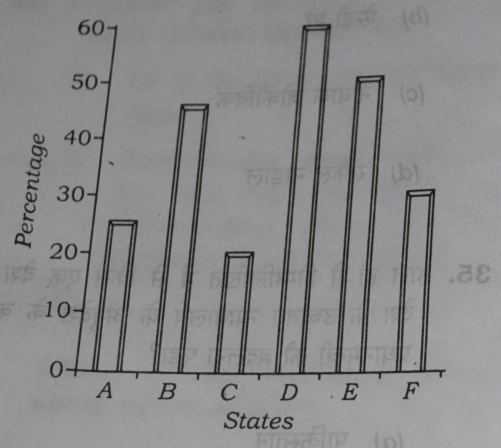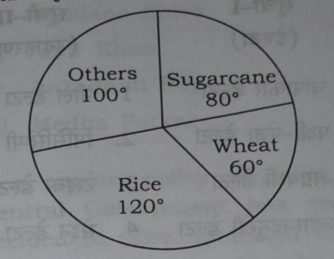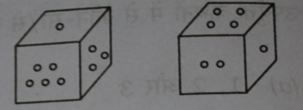## Aptitude/General Intelligence: Internal Breakup

 Maths/Quantitative aptitude 3 Data interpretation 4 Reasoning 13 Total 20

## Maths/Quantitative Aptitude

1. A and B take part in 100 meters race, where A beats B by 10 meters. To favour B, A starts 10 meters behind the starting line in a second 100 meters race, running at their earlier speeds. Which one among the following is true in view of the second race?

1. A and B reach the finishing line simultaneously
2. B beats A by 1 meter
3. B beats A by 2 meters
4. A beats B by 1 meter

## First race

If A finishes the race (100m) in T time, then during T time, B has covered only 90 meters.
Speed x Time=Distance.
Therefore,
Speed of A=100/T
Speed of B=90/T

## Second Race

A has to cover 110 meters @speed 100/T
Time taken by A=(110/100)x T=1.1T
B has to cover only 100 meters @speed 90/T
Time taken by B=(100/90) x T=1.1111…T
So even in second race A is taking less time than B, because 1.1 T is smaller than 1.1111…T
Therefore A finishes the race, quicker than B even in second race. In the light of this information, consider the answer options.

 A and B reach the finishing line simultaneously Not possible, A is finishing race quicker than B. B beats A by 1 meter Not possible, A is finishing race quicker than B B beats A by 2 meters Not possible, A is finishing race quicker than B A beats B by 1 meter we are only left with this option.

2. The average of x, y and z is 30, and the average of x and y is 20. What is the value of z?

1. 10
2. 20
3. 40
4. 50

Total sum of xyz=30*3
Total sum of xy=20*2
So value of Z= 30*3-20*2=50
3. Three dice, whose all six faces are marked ‘1’ to ‘6’, are thrown. The number of ways of getting a sum of 16 is

1. 3
2. 4
3. 6
4. 12

Two dice can give max 6+6=12. So third dice has to give minimum 4
Only two situations possible
First situation: 4+6+6= 16.
Here 3 chairs, but two numbers are same (4,4)
So 3!/2! ways to arrange=3 ways
Second situation 5+6+5
Again 3 chairs but two numbers are same (5,5)
So 3!/2! ways to arrange=3 ways
Total 3+3=6 ways.

## Data Interpretation

Following Histogram shows no. of villages which are not electrifiedFirst tabulate the data

 State % villages not electrified % Villages Electrified=100-previous column A 25 75 B 45 55 C 20 80 D 60 40 E 50 50 F 30 70

1. Which of the following States has twice the percentage of villages electrified in comparison to
State D?
(a) A
(b) C
(c) E
(d) F
State D has 40% villages electrified and State C has 80% villages electrified.
2. How many states have atleast 50% electrified Villages?

1. 1
2. 2
3. 3
4. 5

Atleast 50= 50% or more than 50 percent.
We’ve 6 States, and only one state D doesn’t meet this condition, because it has less than 50% villages electrified.
So 6-1=5 states are atleast 50% villages electrified.

## pie chart

The following two (2) items are based on the given pie-chart which shows the annual agricultural yield of a certain place3. The yield of rice is how much percent more than that of sugarcane?

1. 40%
2. 50%
3. 60%
4. 75%

Rice=120 degrees
Sugarcane=80 degrees
Rice compared to sugar=120/80=3/2=1.5=150%
So Rice’s yield is 50% higher than sugar.
4. If the yield of rice and wheat is 9000 tons, then the yield of excess product of others in comparison with sugarcane is

1. 1000 tons
2. 2000 tons
3. 3000 tons
4. 4000 tons

Rice + Wheat=120+60 degrees=180 degrees=half circle.
If half circle is 9000 tons, then full circle (total production) is 18000 tons.
The question is essentially asking about the difference (D) between production of others and sugar.
The degree difference between others and sugar=100-80=20 degrees.
If 20 degrees=”D” tons
Full circle (360 degrees)=18000 tons
Therefore “D”
=[18000 * 20]/ 360
=50*20
=1000 tons

## Reasoning

1. Which one among the following options is a word that can be formed from the letters used in INTELLIGENCE?

1. SCIENCE
2. NOTICE
3. INCITE
4. ENTAIL

In the word “INTELLIGENCE”, We don’t have “S” so option A is gone
Don’t have “O” os B is gone
Don’t have “A” so D is gone.
Thus we are left with answer (C)
2. Which one among the following options is a word that cannot be formed from the letters used in INTELLIGENCE?

1. ENTICE
2. GENTLE
3. ENGINE
4. INTENT

The word Intent requires two “T”s, But word INTELLIGENCE is providing only one “T”.
3. Two position of the dice are shownWhen 1 is at bottom, which number will be at top?

1. 3
2. 5
3. 6
4. Cannot say,

From first image: number 5 and 3 are not opposite to number 1.
From second image: number 4 and number 2 are not opposite to number 1.
Therefore only “6” is left. So we can deduct that “6” is located on the opposite side of “1”.
Therefore, when 1 is at bottom, 6 will be at top.
Ans (C)

## Clock

4. An accurate clock shows 12 o clock in the noon. through how many degrees will the hour hand rotate when the clock shows 5 o’clock in the same evening.

1. 150
2. 140
3. 125
4. 120

Full clock circle has 360 degrees and 12 “hour gaps”.
So one hour has 360/12 degrees=30 degrees.
So half clock circle (12 noon to 6 evening) has 180 degrees.
So 12 noon to 5 evening = 180 minus 1 hour less gap.
=180-30
=150 degrees
Another approach:
One hour has 360/12 =30 degrees.
12 noon to 5 PM=total 5 hours.
If an hour hand travels 30 degrees in 1 hour
Then in 5 hours, it travels 30×5=150 degrees.

## Calendar-Leap years

5. Consider the following years : 1600, 1700, 1800, 1900, 2000, 2100, 2200, 2300 and 2400 How many leap years are there in the above?

1. None
2. 3
3. 6
4. 9

For century years i.e. those years ending with “00”, you’ve to check divisibility by 400. (not just 4)
If they’re divisible by 400 then they’re leap years.
Accordingly, 1600,2000 and 2400 are leap years.

## Arrangement

6. Ten identical coins are lying on having head ‘H’ face as the upper face. In one attempt, exactly 4 coins can be turned upside down, What is the minimum total number of attempts in which tail ‘T’ face of all the 10 coins can be brought to be the upper face?
(a) 4
(b) 5
(c) 6
(d) 7
It can be done in four moves.
initial situation: H H H H H H H H H H
1ST MOVE: T T T T H H H H H H
2ND MOVE: T T T T H H T T T T
3RD MOVE: T H H H T H T T T T
4TH MOVE: T T T T T T T T T T
(thanks Mr.vijaymani for this)
7. If

1. X is richer than Y
2. Z is richer than X
3. P is richer than Z
4. Q is the richest of all

who among the following will have the central position if they are made to sit in the above degree of richness?

1. X
2. Y
3. Z
4. P

Let’s put them in ascending order

 1 2 3 4 5 Q

According to given statements
X>Y
Z>X
P>Z
Therefore Y<X<Z<P
When we arrange them

 1 2 3 4 5 Y X Z P Q

Z is sitting in the middle chair. So answer is (C)

## Direction Test

8. facing the east, R walks straight 4 km, turns left and walks 3 km, again turns left and walks 4 km. How far is R from the starting point?

1. 2 km
2. 3 km
3. 10 km
4. 11 km

His movement is forming a rectangle of height 3 and width 4. So he is 3 kms away from his starting point.

## Odd men out

9. Which of the following is the odd one?

1. 31
2. 41
3. 51
4. 61

31,41 and 61 are prime numbers. (they donot have any factors except number itself and 1)
51 is not a prime number (because 17×3=51)
Therefore 51 is the odd man here.
10. Missing number in the box?

 3 7 52 5 11 126 ?? 9 107
1. 6
2. 18
3. 26
4. 36

Thanks Kushal for solving this

First Row: 3 + 7*7 = 52
Second Row: 5 + 11*11 = 126
similarly, x + 9*9 = 107
therefore, x = 107-81 = 26

## Assumption –Implied

Codes

1. Only 1 is implied in the statement
2. Only 2 is implied in the statement
3. Both Implied
4. None implied

11. Teaching through TV, is to help student to learn without teachers

1. Students want to study without a teacher
2. Teachers are incapable of teaching.

Ans. None implied. (D). some would say 1 is implied so answer is (A)
12. If you lose after competing hard that is fine. If you lose without trying hard that is what disappoints me.

1. Losing after trying hard is excusable.
2. Losing without effort is regrettable.

Ans. Both implied. (C)